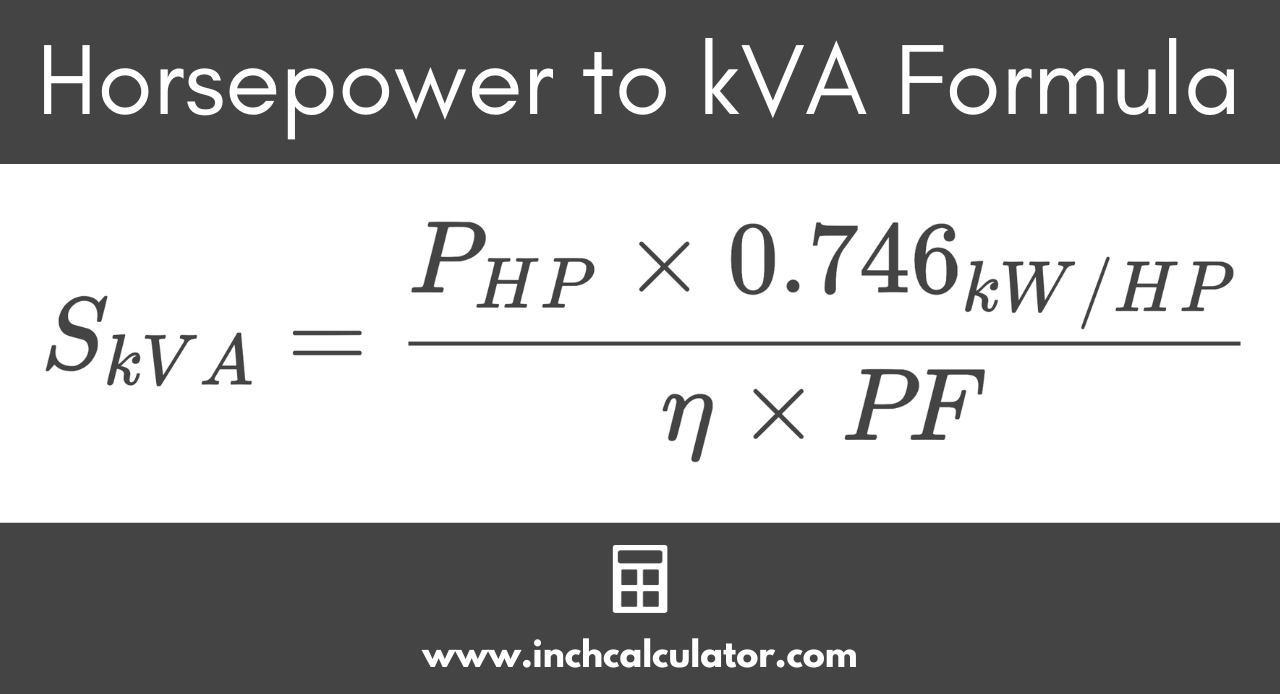# Horsepower to Kilovolt-Amps (kVA) Conversion Calculator

Convert horsepower (HP) to kilovolt-amps (kVA) by entering the power, motor efficiency, and power factor below.

HP
%

## Kilovolt-Amps Results:

kVA
Learn how we calculated this below

## How to Convert Horsepower to kVA

Electrical horsepower is a measure of real power equivalent to 746 watts, while kilovolt-amps measure apparent power. Because power factor and motor efficiency reduce the amount of actual work performed for the amount of apparent power in the circuit, the conversion from horsepower to kVA requires a formula.

### Horsepower to kVA Formula

Use the following formula to convert horsepower to kilovolt-amps.

SkVA = PHP × 0.746 / η × PF

Thus, the apparent power S in kVA is equal to the real power P in horsepower times 0.746 kilowatts per horsepower, divided by the motor efficiency η times the power factor PF.

To convert, substitute the power, efficiency, and power factor values in the formula above and solve. A simpler alternative is to use the calculator above, which does the same thing. If the efficiency is unknown, leave it at 100% for the equation.The formula is derived by following these simple steps.

### Step One: Convert Horsepower to kW

To convert horsepower to kVA, start by multiplying it by 0.746 to convert to kilowatts.

PkW = PHP × 0.746

### Step Two: Account for Motor Efficiency and Power Factor

Next, divide the motor efficiency as a percentage by 100 to get the decimal value, or use a percent to decimal calculator. Then, divide the power in kilowatts by the product of the efficiency and power factor to calculate the apparent power in kilovolt-amps.

SkVA = PkW ÷ (η × PF)

To convert the real power in kilowatts to the apparent power in kilovolt-amps, divide the power in kilowatts by the motor efficiency multiplied by the power factor.

For example, let’s convert 25 horsepower to kVA given a power factor of 0.8 and a motor efficiency of 84%

SkVA = 25 HP × 0.746 / 0.84 × 0.8
SkVA = 18.65 kW / 0.672
SkVA = 27.753 kVA

In this example, 25 horsepower is equal to 27.753 kVA.

## Equivalent Horsepower and kVA Ratings

Equivalent horsepower (HP) and kilovolt-amps (kVA) ratings with an efficiency of 80% and a power factor of 1.
HP kVA
1 HP 0.933 kVA
2 HP 1.87 kVA
3 HP 2.8 kVA
4 HP 3.73 kVA
5 HP 4.66 kVA
6 HP 5.6 kVA
7 HP 6.53 kVA
8 HP 7.46 kVA
9 HP 8.39 kVA
10 HP 9.33 kVA
15 HP 13.99 kVA
20 HP 18.65 kVA
25 HP 23.31 kVA
30 HP 27.98 kVA
35 HP 32.64 kVA
40 HP 37.3 kVA
45 HP 41.96 kVA
50 HP 46.63 kVA
55 HP 51.29 kVA
60 HP 55.95 kVA
65 HP 60.61 kVA
70 HP 65.28 kVA
75 HP 69.94 kVA
80 HP 74.6 kVA
85 HP 79.26 kVA
90 HP 83.93 kVA
95 HP 88.59 kVA
100 HP 93.25 kVA

You might also be interested in our kVA to horsepower calculator.